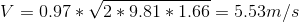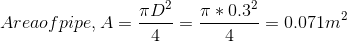# A pitot tube is used to measure the velocity of the water at the center of a pipe (300 mm in diameter). The stagnation pressure head is 6.89 m and the static pressure head is 5.23 m. a. Determine the velocity of water in m/s at the center of the pipe if the coefficient of velocity is 0.97 b. If the velocity is 6.98 m/s, determine the difference of the stagnation pressure head and the static pressure head in meters. Cv = 0.98 c. Determine the coefficient of velocity of the pitot tube if the difference between the stagnation pressure head and the static pressure head in the pipe is 1.89 m. The actual discharge in the pipe is 420 liters/second.

Question-AnswerCategory: Fluid MechanicsA pitot tube is used to measure the velocity of the water at the center of a pipe (300 mm in diameter). The stagnation pressure head is 6.89 m and the static pressure head is 5.23 m. a. Determine the velocity of water in m/s at the center of the pipe if the coefficient of velocity is 0.97 b. If the velocity is 6.98 m/s, determine the difference of the stagnation pressure head and the static pressure head in meters. Cv = 0.98 c. Determine the coefficient of velocity of the pitot tube if the difference between the stagnation pressure head and the static pressure head in the pipe is 1.89 m. The actual discharge in the pipe is 420 liters/second.

A pitot tube is used to measure the velocity of the water at the center of a pipe (300 mm in diameter). The stagnation pressure head is 6.89 m and the static pressure head is 5.23 m.
a. Determine the velocity of water in m/s at the center of the pipe if the coefficient of velocity is 0.97
b. If the velocity is 6.98 m/s, determine the difference of the stagnation pressure head and the static pressure head in meters. Cv = 0.98
c. Determine the coefficient of velocity of the pitot tube if the difference between the stagnation pressure head and the static pressure head in the pipe is 1.89 m. The actual discharge in the pipe is 420 liters/second.

Given
Diameter of pipe, D = 300 mm = 0.3m
Stagnation pressure head, Hp = 6.89 m
Static pressure head, Hs= 5.23 m
a) Central velocity, Vwhere
Cv = Coefficient of velocity = 0.97
g = acceleration due to gravity = 9.81 m/s2
h = Hp – Hs = 6.89 – 5.23 = 1.66 mwhere
V = 6.98 m/s
Cv = Coefficient of velocity = 0.98
g = acceleration due to gravity = 9.81 m/s2
h = Hp – HsSolving,c) Cofficient of velocity, Cv
Given Actual discharge in the pipe, Qa = 420 liters/second = 0.42 m3/s
Diameter of pipe, D = 300 mm = 0.3 mwhere
V = 5.92 m/s
Cv = Coefficient of velocity
g = acceleration due to gravity = 9.81 m/s2
h = 1.89 mSolving